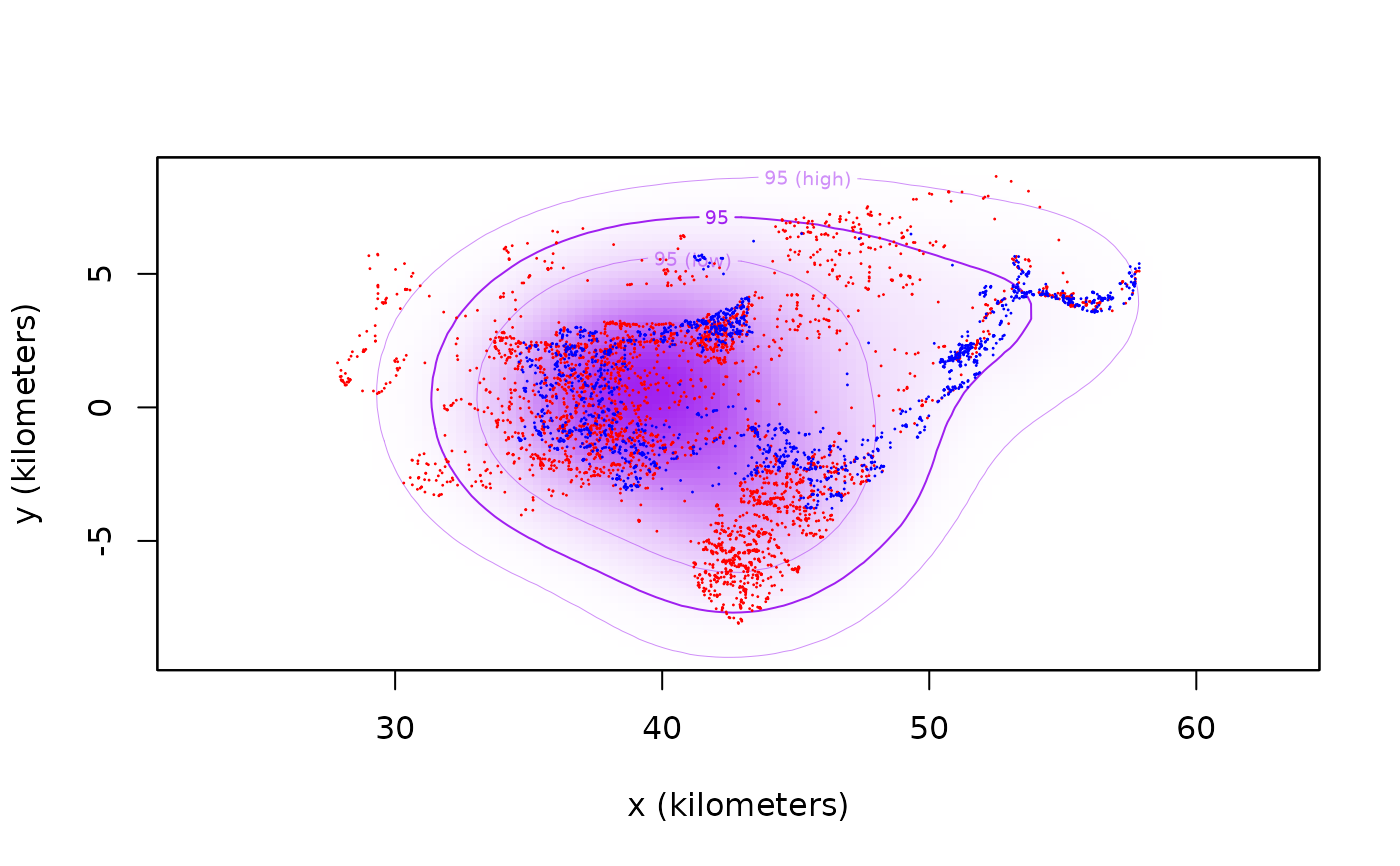Functions to calculate the location distribution of where encounters take place, conditional on said encounters taking place, as described in Noonan et al (2021).

encounter(object,include=NULL,exclude=NULL,debias=FALSE,...)

## Arguments

object A list of aligned UD objects. A matrix of interactions to include in the calculation (see Details below). A matrix of interactions to exclude in the calculation (see Details below). Approximate GRF bias correction (in development). Additional arguments for future use.

## Details

The include argument is a matrix that indicates which interactions are considered in the calculation. By default, include = 1 - diag(length(object)), which implies that all interactions are considered aside from self-interactions. Alternatively, exclude = 1 - include can be specified, and is by-default exclude = diag(length(object)), which implies that only self-encounters are excluded.

## Value

A UD object.

M. J. Noonan, R. Martinez-Garcia, G. H. Davis, M. C. Crofoot, R. Kays, B. T. Hirsch, D. Caillaud, E. Payne, A. Sih, D. L. Sinn, O. Spiegel, W. F. Fagan, C. H. Fleming, J. M. Calabrese, Estimating encounter location distributions from animal tracking data'', Methods in Ecology and Evolution (2021) doi: 10.1111/2041-210X.13597 .

## Author

C. H. Fleming

akde, overlap

## Examples

# \donttest{
library(ctmm)
data(buffalo)

# fit models for first two buffalo
GUESS <- lapply(buffalo[1:2], function(b) ctmm.guess(b,interactive=FALSE) )
# in general, you should use ctmm.select here
FITS <- lapply(1:2, function(i) ctmm.fit(buffalo[[i]],GUESS[[i]]) )
names(FITS) <- names(buffalo[1:2])

# create aligned UDs
UDS <- akde(buffalo[1:2],FITS)

# calculate CDE
CDE <- encounter(UDS)

# plot data and encounter distribution
plot(buffalo[1:2],col=c('red','blue'),UD=CDE,col.DF='purple',col.level='purple',col.grid=NA)
#> DOP values missing. Assuming DOP=1.#> DOP values missing. Assuming DOP=1.# }